# Solving similar triangles 2

##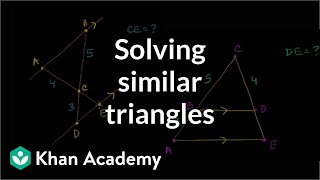By Khan Academy

Sal solves two problems where a missing side length is found by proving that triangles are similar and using this to find the measure.# Solving similar triangles 1

##By Khan Academy

Sal solves two problems where a missing side length is found by proving that triangles are similar and using this to find the measure.# Telling Time to the Minute

##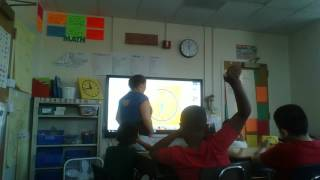By Sabrina brown

the nearest minute and measure time intervals in minutes. Solve word problems involving addition and subtraction of time intervals in minutes##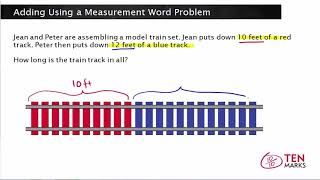By Math mamoth

This lesson presents a measurement word problem that involves addition. A visual is used to write an equation and solve.# Adding and Subtracting Angles: 4.MD.7

##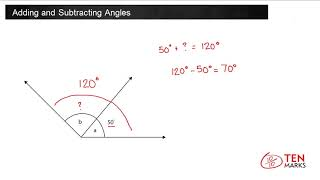By TenmarksAmazon

Recognize angle measure as additive. When an angle is decomposed into non-overlapping parts, the angle measure of the whole is the sum of the angle measures of the parts. Solve addition and subtraction problems to find unknown angles on a diagram in real world and mathematical problems# Find missing angles of triangles

##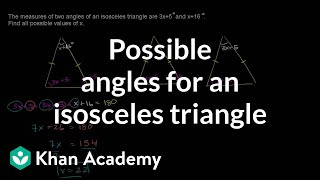By Khan Academy

Sal solves the following problem: The measures of two angles of an isosceles triangle are 3x+5 and x+16. Find all possible values of x.# Elapsed Time on a timeline

##By Skubes ed

Tell and write time to the nearest minute and measure time intervals in minutes. Solve word problems involving addition and subtraction of time intervals in minutes# Unknown angle algebra problems

##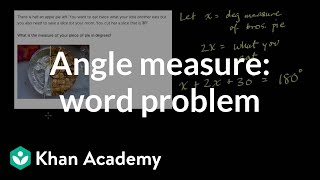By Khan Academy

Solve this word problem to find the measure of angles. In this example you'll split up a pie (don't forget to share!)# Division - Sharing Equally: Grade 3

##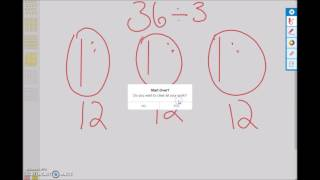By university of Houston mathematics education

Use multiplication and division within 100 to solve word problems in situations involving equal groups, arrays, and measurement quantities, e.g., by using drawings and equations with a symbol for the unknown number to represent the problem.# Division - Sharing Equally: Grade 3

##By university of Houston mathematics education

Use multiplication and division within 100 to solve word problems in situations involving equal groups, arrays, and measurement quantities, e.g., by using drawings and equations with a symbol for the unknown number to represent the problem.# Telling Time to the Nearest Minute

##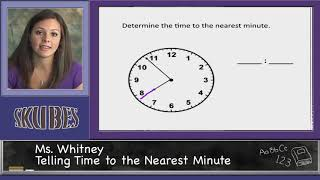By Skubes ed

Tell and write time to the nearest minute and measure time intervals in minutes. Solve word problems involving addition and subtraction of time intervals in minutes, e.g., by representing the problem on a number line diagram.# Division Problem Solving

##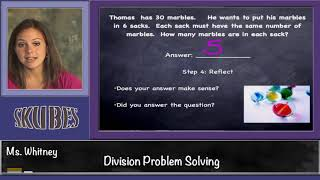By Skubes ed

Use multiplication and division within 100 to solve word problems in situations involving equal groups, arrays, and measurement quantities, e.g., by using drawings and equations with a symbol for the unknown number to represent the problem.1# Significant Digits & Measurements

##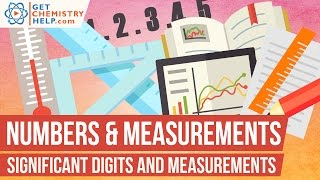By GetChemistryHelp

Significant digits (significant figures, sig figs) indicate the precision of a measurement. This lesson explains how to determine the number of significant digits in a measurement. For practice problems and additional chemistry lessons, visit http://www.getchemistryhelp.com.# Fractions and Line Plots

##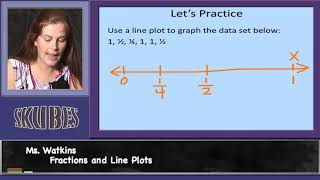By Skubes ed

Use the four operations to solve word problems involving distances, intervals of time, liquid volumes, masses of objects, and money, including problems involving simple fractions or decimals, and problems that require expressing measurements given in a larger unit in terms of a smaller unit.# [5.MD.1-2.0] Convert Standard Measurements - Common Core Standard

##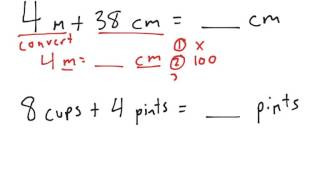By Front Row

Discover more Common Core Math at https://www.frontrowed.com Use measurement conversions in solving multi-step, real world problems
Front Row is a free, adaptive, Common Core aligned math program for teachers and students in kindergarten through eighth grade. Front Row allows students to practice math at their own pace - learning advanced concepts when they're ready and receiving remediation when they struggle. Front Row provides teachers with access to a detailed data dashboard and weekly email reports that show which standards are causing students difficulty, what small groups can be formed for interventions, and how their students are progressing in math.Discover more Common Core Math at https://www.frontrowed.com# Multi Step Equations | MathHelp.com

##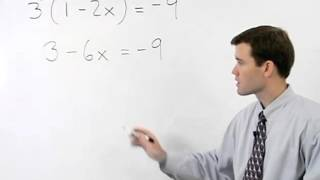By MathHelp.com

This lesson covers complementary angles. Students learn the definition of complementary and supplementary angles, as well as how to find the measures of the complement and supplement of a given angle. Students are also asked to solve word problems in the following form: "If the ratio of an angle to its complement is 2:3, find the measure of each angle."# Ex 2: Set Problem Solving - Find the Number of Element in the Union of Two Sets Using a Formula

##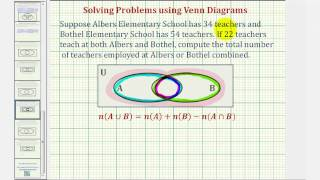By Mathispower4u

Ex 2: Set Problem Solving - Find the Number of Element in the Union of Two Sets Using a Formula# Significant Figures with Counting Numbers and Measurements

##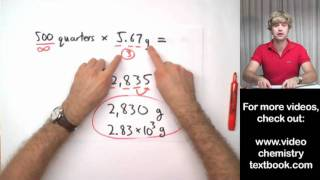By Tyler DeWitt

When and how do you actually use significant figures in math and science problems? Here, we'll look at the different between measurements and counting numbers.# Metric Unit Conversions - YourTeacher.com - Pre Algebra Help

##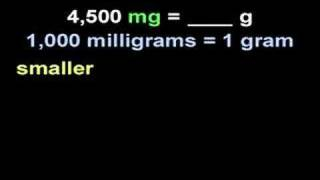By yourteachermathhelp

Students learn that in the metric system all measures of length are based on meters. For example a centimeter is 1/100 of a meter. And all measures of weight are based on grams. For example a kilogram is 1000 grams. And all measures of volume are based on liters. For example a milliliter is 1/1000 of a liter. Students are then asked to solve problems using conversion factors such as 4 500 milligrams = ____ grams.# Understanding Measurement Data Displayed in Line Plots: 2.MD.9

##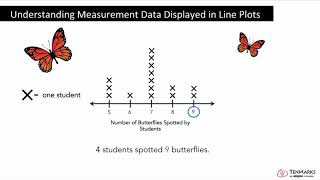By Tenmarks Amazon

Students learn how to interpret measurement data displayed in line plots.